Latest Banking jobs   »   Quantitative Aptitude Quiz For IBPS RRB...

# Quantitative Aptitude Quiz For IBPS RRB PO, Clerk Prelims 2021- 29th April

Q1. If the difference between CI and SI at the end of 2 years is Rs. 100 then what is the principal given that rate is 5% per annum in both the cases and principal is also the same for both cases.
(a) 35000
(b) 40000
(c) 45000
(d) 36000
(e) 32000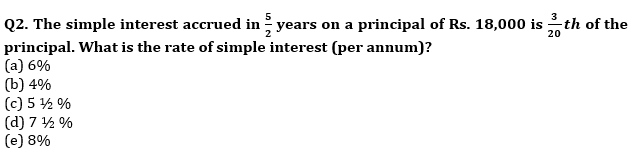Q3. Rahul invested for 2 more months than the time for which Karan invested in the same business. If Karan invested Rs.3500 for 10 months then find the money invested by Rahul, such that ratio of annual profit of Rahul to Karan is 9 : 7.
(a) Rs. 4500
(b) Rs. 4200
(c) Rs. 4350
(d) Rs. 3750
(e) None of these

Q4. Kaushal invested Rs.800 at SI for 2 years. If he had invested this amount at CI at same rate of interest, he would receive Rs. 32 more as an interest amount. Find rate of interest per annum.
(a) 16%
(b) 20%
(c) 10%
(d) 15%
(e) None of these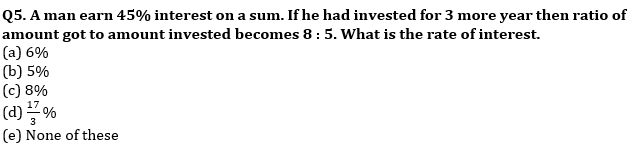Q6. X and Y started a business with Rs. 8000 and Rs. 5000 respectively. After some months, Y left the business. If at the end of a year, X share of profit is Rs.3000 out of total profit of Rs. 4250, then find after how many months ‘Y’ leave the business?
(a) 6 months
(b) 7 months
(c) 8 months
(d) 10 months
(e) None of these

Q7. C.I. and S.I. for a certain sum at certain rate of interest for 2 years is Rs. 440 and Rs. 400 respectively. Find the principal?
(a) Rs. 2000
(b) Rs. 1000
(c) Rs. 1600
(d) Rs. 1200
(e) Rs. 500

Q8. In a business, total investment of P, Q and R is Rs. 74000. P invest Rs. 8000 more than Q and Q invest Rs. 6000 more than R. All three invested for same time. If total profit is Rs. 44,400 then find the profit share of Q?
(a) Rs. 13,200
(b) Rs. 14,400
(c) Rs. 9,600
(d) Rs. 36,000
(e) Rs. 7,200

Q9. A sum Rs. 1800 partly lent at 7% p.a. and partly at 9% p.a. at S.I. for three years and earned a total interest of Rs. 420. Find ratio of sum invested at 7% to sum invested at 9% ?
(a) 11 : 7
(b) None of these
(c) 9 : 7
(d) 7 : 11
(e) 7 : 5
QCreator Deepak

Q10. A man invested Rs. X at the rate of 20% p.a. on simple interest for three year and get total interest of Rs. 1020. If man invested same amount at the rate of 10% p.a. on compound interest, then how much amount man will get after three years?
(a) 2278.7 Rs.
(b) 2298.7 Rs.
(c) 2288.7 Rs.
(d) 2262.7 Rs.
(e) 2290.7 Rs.

Q11. Veer and Mohit invested total Rs. 1750 in a business, while Veer invested for 8 months and Mohit invested for 10 months. If the get profit in the ratio of 16 : 15, then find the investment of Mohit?
(a) 850 Rs.
(b) 950 Rs.
(c) 1050 Rs.
(d) 750 Rs.
(e) 1250 Rs.

Q12. Amount Invested by ‘Bhavya’ is 3.5 times of amount invested by ‘Veer’ in a business and ratio of time period for ‘Veer’ & Bhavya’ investment is 3 : 1. If total investment of ‘Veer’ and ‘Bhavya’ together is Rs. 54000 and profit share of Veer is 2400 less than his investment, then find the difference between profit share of ‘Veer’ and ‘Bhavya’?
(a) Rs.1350
(b) Rs. 1500
(c) Rs.1650
(d) Rs. 1850
(e) Rs. 1600

Q13. A and B enter into a partnership business with total investment of Rs. 12000 and time period for which they invested is in the ratio of 8 : 5 respectively. If ratio of profit share of A & B is 8 : 7, then find investment of B?
(a) 5000 Rs.
(b) 8000 Rs.
(c) 9600 Rs.
(d) 7000 Rs.
(e) 10800 Rs.

Q14. A man invested Rs. 1200 on simple interest at rate of 10% p.a. for two years. If he invested Rs. X more at the same rate and for same period of time on compound interest, then he will get Rs. 96 more as interest. Find value of X?
(a) 400 Rs.
(b) 350 Rs.
(c) 450 Rs.
(d) 550 Rs.
(e) 420 Rs.

Q15. A sum of Rs. 1,35,000 is divided into three parts and the corresponding interest earned after 3 years, 6 years and 9 years may be equal. If the investment is based on simple interest are 4%, 6% and 8% respectively. What is difference between the sum of highest and smallest sum?
(a) Rs. 75,000
(b) Rs. 80,000
(c) Rs. 77,000
(d) Rs. 55,000
(e) Rs. 70,000

Practice More Questions of Quantitative Aptitude for Competitive Exams:

###### Study Plan for IBPS RRB PO/Clerk Prelims 2021

Solutions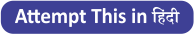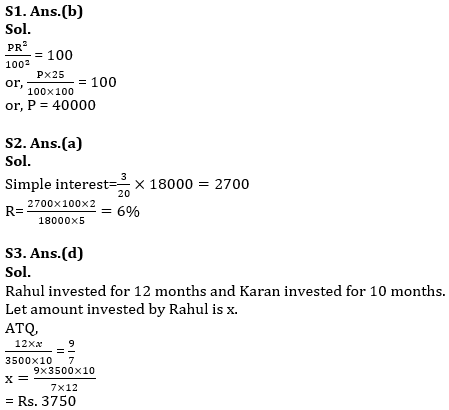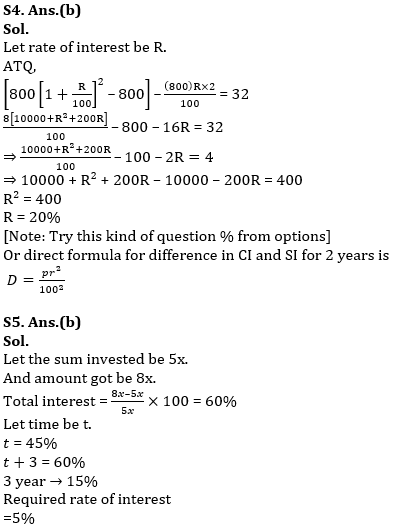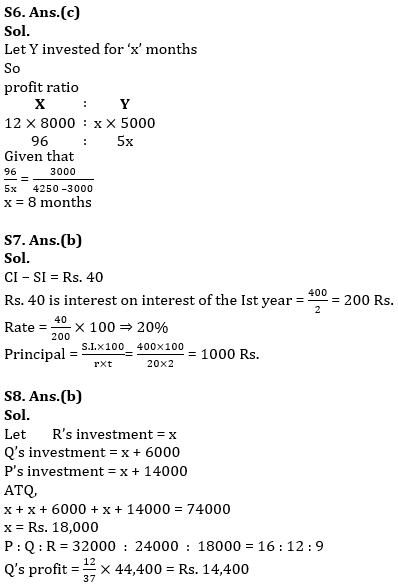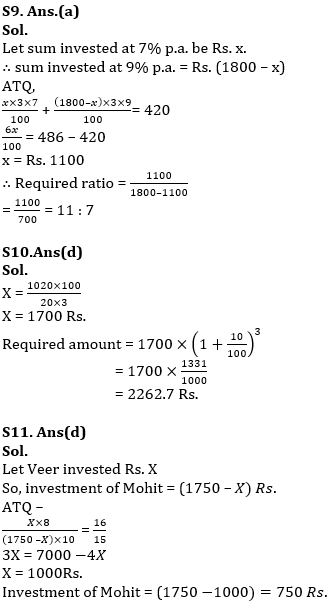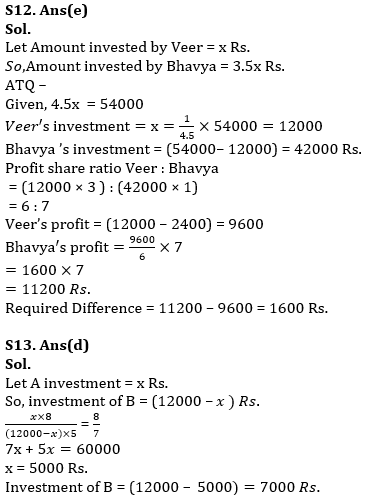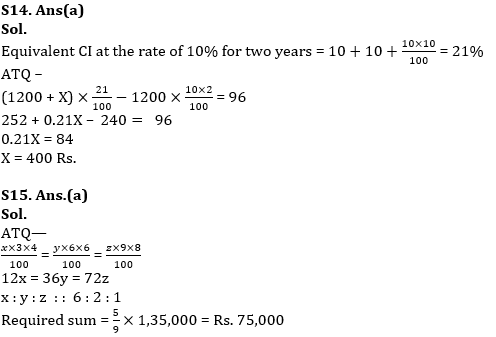×

Thank You, Your details have been submitted we will get back to you.Join India's largest learning destination

What You Will get ?

•Job Alerts
•Daily Quizzes
•Subject-Wise Quizzes
•Current Affairs
•Previous year question papers
•Doubt Solving session

ORJoin India's largest learning destination

What You Will get ?

•Job Alerts
•Daily Quizzes
•Subject-Wise Quizzes
•Current Affairs
•Previous year question papers
•Doubt Solving session

ORJoin India's largest learning destination

What You Will get ?

•Job Alerts
•Daily Quizzes
•Subject-Wise Quizzes
•Current Affairs
•Previous year question papers
•Doubt Solving session

Enter the email address associated with your account, and we'll email you an OTP to verify it's you.Join India's largest learning destination

What You Will get ?

•Job Alerts
•Daily Quizzes
•Subject-Wise Quizzes
•Current Affairs
•Previous year question papers
•Doubt Solving session

Enter OTP

Please enter the OTP sent to
/6

Did not recive OTP?

Resend in 60sJoin India's largest learning destination

What You Will get ?

•Job Alerts
•Daily Quizzes
•Subject-Wise Quizzes
•Current Affairs
•Previous year question papers
•Doubt Solving sessionJoin India's largest learning destination

What You Will get ?

•Job Alerts
•Daily Quizzes
•Subject-Wise Quizzes
•Current Affairs
•Previous year question papers
•Doubt Solving session

Almost there

+91Join India's largest learning destination

What You Will get ?

•Job Alerts
•Daily Quizzes
•Subject-Wise Quizzes
•Current Affairs
•Previous year question papers
•Doubt Solving session

Enter OTP

Please enter the OTP sent to Edit Number

Did not recive OTP?

Resend 60

By skipping this step you will not recieve any free content avalaible on adda247, also you will miss onto notification and job alerts

Are you sure you want to skip this step?

By skipping this step you will not recieve any free content avalaible on adda247, also you will miss onto notification and job alerts

Are you sure you want to skip this step?